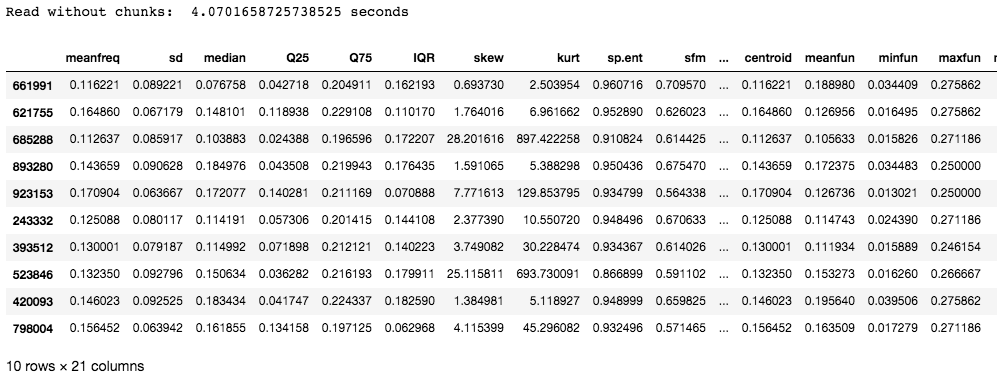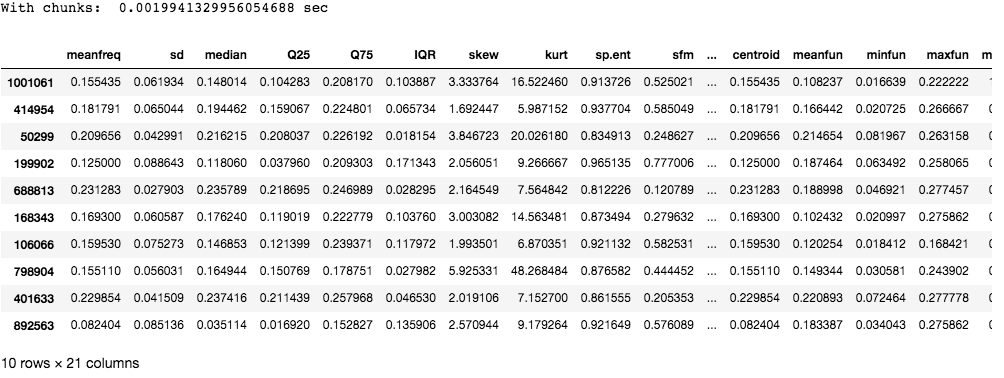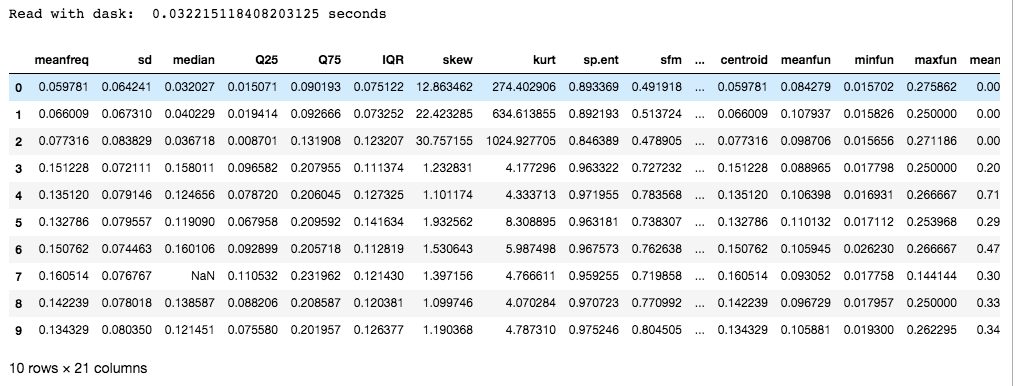# Working with large CSV files in Python

Data plays a key role in building machine learning and the AI model. In today’s world where data is being generated at an astronomical rate by every computing device and sensors, it is important to handle huge volumes of data correctly. One of the most common ways of storing data is in the form of Comma-Separated Values(CSV). Directly importing a large amount of data leads to out-of-memory error and reading the entire file at once leads to system crashes due to insufficient RAM.

The following are few ways to effectively handle large data files in .csv format. The dataset we are going to use is gender_voice_dataset.

One way to process large files is to read the entries in chunks of reasonable size, which are read into the memory and are processed before reading the next chunk. We can use the chunk size parameter to specify the size of the chunk, which is the number of lines. This function returns an iterator which is used to iterate through these chunks and then processes them. Since only a part of the file is read at a time, low memory is enough for processing.

The following is the code to read entries in chunks.

`chunk = pandas.read_csv(filename,chunksize=...)`

Below code shows the time taken to read a dataset without using chunks:

## Python3

 `# import required modules ` `import` `pandas as pd ` `import` `numpy as np ` `import` `time ` ` `  `# time taken to read data ` `s_time ``=` `time.time() ` `df ``=` `pd.read_csv(``"gender_voice_dataset.csv"``) ` `e_time ``=` `time.time() ` ` `  `print``(``"Read without chunks: "``, (e_time``-``s_time), ``"seconds"``) ` ` `  `# data ` `df.sample(``10``) `

Output:The data set used in this example contains 986894 rows with 21 columns. The time taken is about 4 seconds which might not be that long, but for entries that have millions of rows, the time taken to read the entries has a direct effect on the efficiency of the model.

Now, let us use chunks to read the CSV file:

## Python3

 `# import required modules ` `import` `pandas as pd ` `import` `numpy as np ` `import` `time ` ` `  `# time taken to read data ` `s_time_chunk ``=` `time.time() ` `chunk ``=` `pd.read_csv(``'gender_voice_dataset.csv'``, chunksize``=``1000``) ` `e_time_chunk ``=` `time.time() ` ` `  `print``(``"With chunks: "``, (e_time_chunk``-``s_time_chunk), ``"sec"``) ` `df ``=` `pd.concat(chunk) ` ` `  `# data ` `df.sample(``10``) `

Output:As you can see chunking takes much lesser time compared to reading the entire file at one go.

Dask is an open-source python library that includes features of parallelism and scalability in Python by using the existing libraries like pandas, NumPy, or sklearn.

To install:

`pip install dask`

## Python3

 `# import required modules ` `import` `pandas as pd ` `import` `numpy as np ` `import` `time ` `from` `dask ``import` `dataframe as df1 ` ` `  `# time taken to read data ` `s_time_dask ``=` `time.time() ` `dask_df ``=` `df1.read_csv(``'gender_voice_dataset.csv'``) ` `e_time_dask ``=` `time.time() ` ` `  `print``(``"Read with dask: "``, (e_time_dask``-``s_time_dask), ``"seconds"``) ` ` `  `# data ` `dask_df.head(``10``) `

Output:Dask is preferred over chunking as it uses multiple CPU cores or clusters of machines (Known as distributed computing). In addition to this, it also provides scaled NumPy, pandas, and sci-kit libraries to exploit parallelism.

Note: The dataset in the link has around 3000 rows. Additional data was added separately for the purpose of this article, to increase the size of the file. It does not exist in the original dataset.

Whether you're preparing for your first job interview or aiming to upskill in this ever-evolving tech landscape, GeeksforGeeks Courses are your key to success. We provide top-quality content at affordable prices, all geared towards accelerating your growth in a time-bound manner. Join the millions we've already empowered, and we're here to do the same for you. Don't miss out - check it out now!

Previous
Next